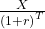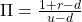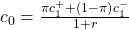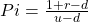Basics of Derivative Pricing and Valuation | IFT World
IFT Notes for Level I CFA® Program
IFT Notes for Level I CFA® Program

# Part 5

## 10. Put-Call Parity, Put-Call-Forward Parity

The four instruments in a put-call parity are as follows:

• Asset: An asset such as a stock with no carrying costs and no interim cash flows. At time 0, the price of the stock is S0
• Zero-coupon bond: A zero-coupon bond with a face value of X that matures at time T. At time 0, the value of this bond is.
• Call option: A call option on the stock with a strike price of X, expires at time T. Sis the underlying price at expiration T.
• Put option: A put option on the stock with a strike price of X, expires at time T.

Note: the strike price X of the options is the same as the par value of the bond.

According to put-call parity, fiduciary call = protective put.

A protective put is like buying insurance for an asset you own, specifically a stock, to protect against a downside. A protective put is to buy the underlying and buy a put option on it. For instance, you own 1,000 shares of Apple, the market is highly volatile and you are worried about a huge decline in the near term. To limit the downside risk, you can buy a put option on the stock by paying a premium. This is called a protective put.

Interpretation:

• As you can see, the downside risk is limited to the premium paid for the put. If the underlying price falls below X, it can be still be sold at X by exercising the put option.
• If the underlying rises above X, then it can be sold at the market price and the put option expires worthless.

A fiduciary call is a long call plus a risk-free bond. The diagram below shows the payoff for a fiduciary call:

The table below shows how the fiduciary call is equal to the protective put under two possible scenarios: when the stock is above the exercise price (call is in-the-money) and the stock is below the exercise price (put is in-the-money).

 Outcome at time T when: → Put expires in-the-money (ST < X) Call expires in-the-money (ST > = X) Protective put Asset ST 0 Long puts X-ST 0 Total X ST Fiduciary call Long call 0 ST – X Risk-free bond X X Total X ST

 Fiduciary Call Protective Put Constituents Long call + risk-free bond Long put + stock Equation c0+p0+S0 Payoff at T if call expires in the money (ST > = X) ST ST Payoff at T if put expires in the money (ST < X) X X Easy way to remember (alphabetical order BCPS) B + C P + S Put-call parity: c0+= p0 + S0

If at time 0, the fiduciary call is not priced the same as the protective put, then there is an arbitrage opportunity. The put-call parity relationship can be rearranged in the following ways:

Synthetic call: c0 = p0 + S0 –Synthetic bond:= p0 + S0 – c0
Synthetic stock: S0 = c0+– p0
Synthetic put: p= c0+– S0

### 10.1 Put-Call Forward Parity

In this section, we see how to create protective put with a forward contract. Recall we saw in an earlier section that:

Asset – Forward = Risk-free Bond (long asset + short forward = risk-free bond)

Rearranging, we get: Asset = Forward + risk-free bond

Now, if we substitute the asset part in a protective put with the above equation, then we get:

Protective put: Asset + put = Forward + risk-free bond + put

The exhibit below shows that the payoff is the same for a protective put with an asset as well as a forward contract when the put expires in and out of the money.

 Outcome at T Put Expires In the Money  (ST < X) Put Expires Out of the Money (ST >= X) Protective put with asset Asset ST ST Long put X – ST 0 Total X ST Protective put with forward contract Risk-free bond Fo(T) Fo(T) Forward contract ST – Fo(T) ST – Fo(T) Long put X – ST 0 Total X ST

Source: CFA Program Curriculum, Basics of Derivative Pricing and Valuation

Interpretation:

• The risk-free bond has a par value equal to the forward price of F0 (T).
• Value of the forward contract at expiration = ST – F0 (T).
• Notice that the value of the forward contract at expiration is the same irrespective of whether the put expires in or out of the money.

According to put-call parity, since a fiduciary call = protective put, a fiduciary call must be equal to a protective put with a forward contract.

Bond + Call = Long Put + Asset

Replacing S in the above equation with a forward contract, we get:

Bond + Call = Long Put + Long forward + Risk-free bond

The exhibit below shows that the payoffs for fiduciary call is equal to protective put when call and put expire in the money.

 Outcome at T Put Expires In the Money (ST < X) Put Expires Out of the Money (ST >= X) Protective put with forward contract Risk-free bond Fo(T) Fo(T) Forward contract ST – Fo(T) ST – Fo(T) Long put X – ST 0 Total X ST Fiduciary call Call 0 ST – X Risk-free bond X X Total X ST

## 11. Binomial Valuation of Options

This section deals with the payoff of an option. The payoff of an option depends on the value of the underlying at expiration, which cannot be known with certainty ahead of time. What matters from an option’s perspective is whether it expires in or out of the money, i.e., whether the stock price was above or below the strike price at expiration.

The binomial model is a simple model for valuing options based on only two possible outcomes for a stock’s movement: going up and going down. The exhibit below shows the binomial option-pricing model.

Source: CFA Program Curriculum, Basics of Derivative Pricing and Valuation

The notations used in the model are explained below:

 Notation Explanation S0 Value of the stock at time t = 0 C0 Price of the call option at time t = 0 S1+ Price of the stock at time t = 1; + indicates the price of the stock went up S1– Price of the stock at time t = 1; – indicates the price of the stock went down c1+ Value of the call option at t = 1 c1– Value of the call option at t = 1 u Up factor = S1+/S0 D Down factor = S1–/S0

Π and 1- π are called the synthetic probabilities; they represent the weighted average of producing the next two possible call values, a type of expected future value. By discounting the future expected call values at the risk-free rate, we can get the current call value.

Risk-neutral probabilitywhere:
r = risk-free rate
u = up factor; d = down factor

Let us do a numerical example to calculate the call price using a 1 – period binomial model now. Data already given to us is in normal font, while the calculated ones are in bold.

The value of the call option at time 0 using the 1-period binomial model is 6.35.

Call price using the binomial modelwhere:

π = risk-neutral probability

r = risk-free rate

c0 = price of call option at time 0

Some important points about the binomial pricing model:

1. Volatility of the underlying: The volatility of the underlying is important in determining the value of the option. It is shown in the difference between S1+ and S1. The higher the volatility, the greater the difference between the stock prices S1+ and S1,and c1+ and c1.
2. The probabilities of the up and down moves do not appear in the formula.
3. Instead, what is used is the synthetic or pseudo probability, π, to determine the expected future value.4. The formula for calculating c0is the expected future value discounted at the risk-free rate.

Binomial Value of Put Options

What is the value of the put option using the same principle discussed in the previous section?

Assume the following data is given:

S0 = 40; u = 1.2; d= 0.75; X = 38; r = 5%; p0 =?

The value of the put option at time 0 using the 1-period binomial model is 2.531.

NEW FREE 2023 CFA® Exam Prep Courses. Get One Full Topic for Free!
This is default text for notification bar